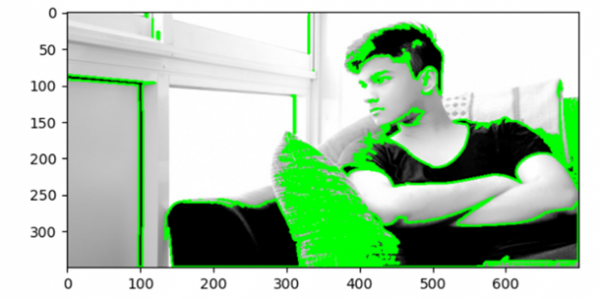# Detecting contours in an image using OpenCV

In this program, we will detect contours in an image. Contours can be explained simply as a curve joining all the continuous points having the same color or intensity. The contours are a useful tool for shape analysis and object detection and recognition.

## Algorithm

Step 1: Import OpenCV.
Step 2: Import matplotlib.
Step 4: Convert the image from bgr2rgb.
Step 5: Convert the rgb image to grayscale.
Step 4: Perform thresholding on the image.
Step 5: Find contours on the image.
Step 6: Draw contours on the image.
Step 7: Display the output.

## Example Code

import cv2
import matplotlib.pyplot as plt

image = cv2.cvtColor(image, cv2.COLOR_BGR2RGB)
gray = cv2.cvtColor(image, cv2.COLOR_RGB2GRAY)
ret, binary = cv2.threshold(gray, 127,255, cv2.THRESH_BINARY_INV)
contours, hierarchy = cv2.findContours(binary, cv2.RETR_TREE, cv2.CHAIN_APPROX_SIMPLE)

image = cv2.drawContours(image, contours, -1, (0,255,0), 2)
plt.imshow(image)
plt.show()

## Output